#### The general framework

The framework is usually applied to infinite-horizon problems under probabilistic uncertainty. The discounted-cost model is most popular; however, we will mostly work with Formulation 10.1 because it is closer to the main theme of this book. It has been assumed so far that when planning under Formulation 10.1, all model components are known, including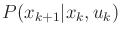. This can be considered as a traditional framework, in which there are three general phases:

1. [] Learning phase: The transition probabilities are estimated by visiting states in, trying actions, and gathering statistics. When this phase concludes, the model of the environment is completely known.
2. [] Planning phase: An algorithm computes a feedback plan using a method such as value iteration or policy iteration.
3. [] Execution phase: The plan is executed on a machine that is connected to the same environment on which the learning phase was applied.

The simulation-based framework combines all three of these phases into one. Learning, planning, and execution are all conducted by a machine that initially knows nothing about the state transitions or even the cost terms. Ideally, the machine should be connected to a physical environment for which the Markov model holds. However, in nearly all implementations, the machine is instead connected to a Monte Carlo simulator as shown in Figure 10.11. Based on the current state, the algorithm sends an action,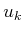, to the simulator, and the simulator computes its effect by sampling according to its internal probability distributions. Obviously, the designer of the simulator knows the transition probabilities, but these are not given directly to the planning algorithm. The simulator then sends the next state,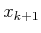, and cost,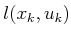, back to the algorithm.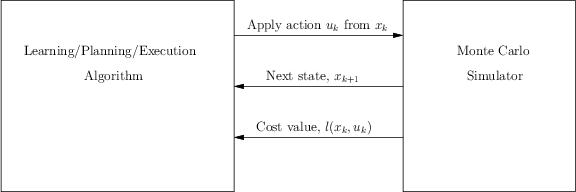For simplicity,is used instead of allowing the cost to depend on the particular nature action, which would yield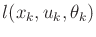. The explicit characterization of nature is usually not needed in this framework. The probabilitiesare directly learned without specifying nature actions. It is common to generalize the cost term fromto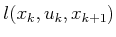, but this is avoided here for notational convenience. The basic ideas remain the same, and only slight variations of the coming equations are needed to handle this generalization.

The simulator is intended to simulate reality,'' in which the machine interacts with the physical world. It replaces the environment in Figure 1.16b from Section 1.4. Using the interpretation of that section, the algorithms presented in this context can be considered as a plan as shown in Figure 1.18b. If the learning component is terminated, then the resulting feedback plan can be programmed into another machine, as shown in Figure 1.18a. This step is usually not performed, however, because often it is assumed that the machine continues to learn over its lifetime.

One of the main issues is exploration vs. exploitation . Some repetitive exploration of the state space is needed to gather enough data that reliably estimate the model. For true theoretical convergence, each state-action pair must be tried infinitely often. On the other hand, information regarding the model should be exploited to efficiently accomplish tasks. These two goals are often in conflict. Focusing too much on exploration will not optimize costs. Focusing too much on exploitation may prevent useful solutions from being developed because better alternatives have not yet been discovered.

Steven M LaValle 2020-08-14